# Maximum Power Transfer Theorem | Proof,Formula

Maximum power transfer theorem is a tool for determining the condition of maximum power transfer. Maximum power transfer theorem states that the source can deliver maximum power to load if the load resistance is equal to the source resistance. The maximum power transfer theorem is applicable for AC and DC circuits. To obtain the maximum power from the source, load resistance should be equal to the source resistance. We will derive the mathematical proof of maximum power transfer theorem and efficiency of the system under maximum power transfer.

## Explanation of Maximum Power Transfer Theorem

In case of DC source, it  has certain resistance. In case of AC source it has certain impedance. The source feeds current to the load. The voltage drop takes place in the source impedance when current flows through the circuit.The power lost in the source resistance must be minimum to get the maximum power delivery of the source.

If the power loss in the source resistance is more, the maximum power delivery from source can not be ensured. There is certain relationship between the source impedance and load impedance for which maximum power delivery from the source can be achieved. The maximum power theorem states that if the load resistance is equal to the source resistance, the maximum power will be delivered by the source.

The source resistance is the resistance viewed from the load side, and we can find its value using the  Thevenin theorem. Therefore, the source resistance is also called Thevenin resistance. We take DC circuit to understand the maximum power transfer theorem.

### Thevenins Equivalent CircuitIn the above Thevenin Equivalent  circuit, the maximum power transfer theorem states that “the maximum amount of power will be delivered in the load resistance if  the load resistance is equal to the  the Thevenin or Norton source resistance.

The source resistance viewed from the load side is Rth and the Thevenin voltage is V0. According to maximum power transfer theorem, the maximum power transfer occurs when the source resistance is equal to the load resistance.

## Maximum Power Transfer Example – 1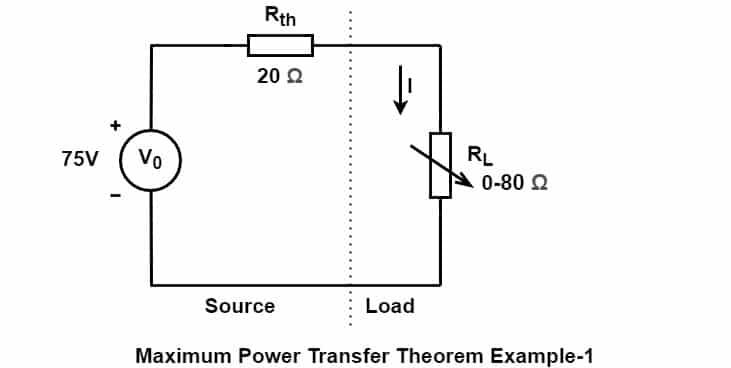According to maximum power transfer theorem the source delivers maximum power to load if the value of load resistance is equal to the source resistance. The value of the load resistance should be 20 Ω.  Now let us verify this statement by calculating the power consumed by the load at various load settings.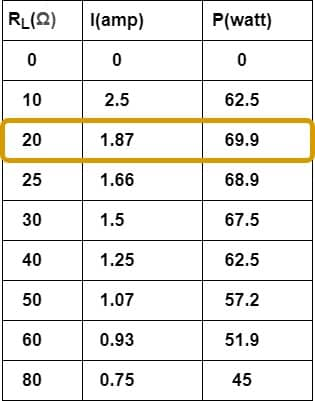## Proof of Maximum Power Transfer Theorem

We take the following DC circuit to derive the condition of maximum power transferThe DC source supply current to the load. The power dissipation in the load resistor RL is P= I2R. The current I flowing through the  circuit is:

Equation(3) can be solved as follows.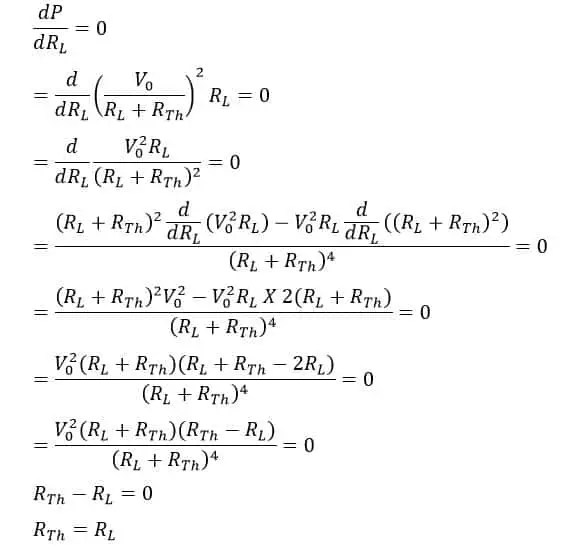Thus, the condition for Maximum Power Transfer  is  the source delivers maximum power to load, if the source resistance is equal to the load resistance. Now , we will calculate the system efficiency  under maximum power transfer condition.

## Efficiency of Maximum Power Transfer

To calculate the efficiency, we first calculate the maximum power transfer when the load resistance is equal to the source resistance.

Maximum Power Delivered to the Load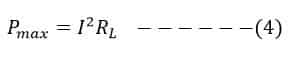Total power transferred from source is:We can calculate the maximum efficiency  by Dividing equation(4) and equation(5)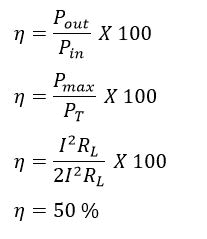Hence, at the condition of maximum power transfer the efficiency is 50%. At maximum power transfer condition, the source delivers 50 % of the generated power to the load, and at other conditions the source drivers small percentage of power to the load.### Application of  Maximum Power Transfer Theorem

The efficiency of the electrical system is 50 %  when the load resistance is equal to the source resistance. It means source deliver 50 % power to the load and remaining 50% power lost in the circuit. The MPTT is not applicable where large power transfer is taking place.In view of this MPTT is not applicable for power transmission. In communication circuit, the magnitude of power transfer is very small and low efficiency is not a problem in communication circuit. The maximum power transfer theorem has tremendous applications in communication circuit for impedance matching.
Related posts

### 2 thoughts on “Maximum Power Transfer Theorem | Proof,Formula”

1.Wow. You described well. Especially that tabular example.